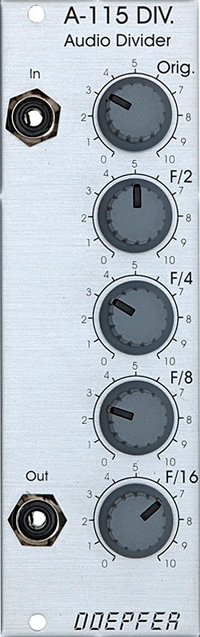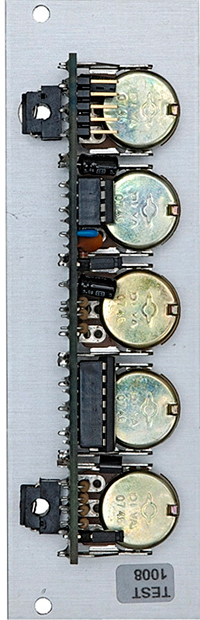doepfer a-115 audio divider price : \$80.00module a-115 is a four-way frequency divider. the frequency of a signal at the input is halved (half frequency = first sub-octave), quartered (1/4 frequency = second sub-octave), and so on. in this way, the divider produces four sub-octaves (f/2 down to f/16).at the output, the a-115 produces a summed mix of the original and the four sub-octaves. there are attenuators to control the amount (ie. amplitude) of the original signal and each of the sub-octaves.

bear in mind that the sub-octaves output by the a-115 are all true square waves. at the output there are always four square waves and the original signal available.this module has a maximum current draw of 20ma. it requires 8 te/hp worth of space to fit in a eurorack frame.